# Phi_K basic tutorial¶

This notebook guides you through the basic functionality of the phik package. The package offers functionality on three related topics:

1. Phik correlation matrix
2. Significance matrix
3. Outlier significance matrix

For more information on the undertlaying theory, the user is referred to our paper.

In :
# import standard packages
import numpy as np
import pandas as pd
import matplotlib.pyplot as plt
import itertools

import phik

from phik import resources
from phik.binning import bin_data
from phik.report import plot_correlation_matrix

%matplotlib inline

In :
# if one changes something in the phik-package one can automatically reload the package or module


A simulated dataset is part of the phik-package. The dataset concerns fake car insurance data. Load the dataset here:

In :
data = pd.read_csv( resources.fixture('fake_insurance_data.csv.gz') )


# Take a first look at the data¶

Let's use a simple data.head() to get an idea of what the data looks like and inspect the different types of variables.

In :
data.head()

Out:
car_color driver_age area mileage car_size
0 black 26.377219 suburbs 156806.288398 XXL
1 black 58.976840 suburbs 74400.323559 XL
2 multicolor 55.744988 downtown 267856.748015 XXL
3 metalic 57.629139 downtown 259028.249060 XXL
4 green 21.490637 downtown 110712.216080 XL

# Specify bin types¶

The phik-package offers a way to calculate correlations between variables of mixed types. Variable types can be inferred automatically although we recommend variable types to be specified by the user.

Because interval type variables need to be binned in order to calculate phik and the signifiance, a list of interval variables is created.

In :
data_types = {'severity': 'interval',
'driver_age':'interval',
'satisfaction':'ordinal',
'mileage':'interval',
'car_size':'ordinal',
'car_use':'ordinal',
'car_color':'categorical',
'area':'categorical'}

interval_cols = [col for col, v in data_types.items() if v=='interval' and col in data.columns]
interval_cols

Out:
['driver_age', 'mileage']

# Visually inspect pairwise correlations¶

## Bin the interval variables¶

To get a feeling for the data, let's bin the interval variables and create 2d histograms to inspect the correlations between variables. By binning the interval variables we can treat all variable types in the same way.

In :
# bin the interval variables
data_binned, binning_dict = bin_data(data, cols=interval_cols, retbins=True)

In :
binning_dict

Out:
{'driver_age': [(18.030700817879673, 28.34523864121504),
(28.34523864121504, 38.659776464550404),
(38.659776464550404, 48.974314287885775),
(48.974314287885775, 59.288852111221146),
(59.288852111221146, 69.60338993455652),
(69.60338993455652, 79.91792775789187),
(79.91792775789187, 90.23246558122725),
(90.23246558122725, 100.54700340456262),
(100.54700340456262, 110.86154122789799),
(110.86154122789799, 121.17607905123334)],
'mileage': [(53.54305708442213, 30047.010932306846),
(30047.010932306846, 60040.478807529274),
(60040.478807529274, 90033.94668275169),
(90033.94668275169, 120027.41455797412),
(120027.41455797412, 150020.88243319656),
(150020.88243319656, 180014.35030841897),
(180014.35030841897, 210007.81818364142),
(210007.81818364142, 240001.28605886383),
(240001.28605886383, 269994.75393408624),
(269994.75393408624, 299988.2218093087)]}
In :
# plot each variable pair
plt.rc('text', usetex=False)

n=0
for i in range(len(data.columns)):
n=n+i

ncols=3
nrows=int(np.ceil(n/ncols))
fig, axes = plt.subplots(nrows, ncols, figsize=(15,4*nrows))
ndecimals = 0

for i, comb in enumerate(itertools.combinations(data_binned.columns.values, 2)):

c = int(i%ncols)
r = int((i-c)/ncols )

# get data
c0, c1 = comb
datahist = data_binned.groupby([c0,c1])[c0].count().to_frame().unstack().fillna(0)
datahist.columns = datahist.columns.droplevel()

# plot data
img = axes[r][c].pcolormesh(datahist.values, edgecolor='w', linewidth=1)

# axis ticks and tick labels
if c0 in binning_dict.keys():
ylabels = ['{1:.{0}f}_{2:.{0}f}'.format(ndecimals, binning_dict[c0][i], binning_dict[c0][i])
for i in range(len(binning_dict[c0]))]
else:
ylabels = datahist.index

if c1 in binning_dict.keys():
xlabels = ['{1:.{0}f}_{2:.{0}f}'.format(ndecimals, binning_dict[c1][i], binning_dict[c1][i])
for i in range(len(binning_dict[c1]))]
else:
xlabels = datahist.columns

# axis labels
axes[r][c].set_yticks(np.arange(len(ylabels)) + 0.5)
axes[r][c].set_xticks(np.arange(len(xlabels)) + 0.5)
axes[r][c].set_xticklabels(xlabels, rotation='vertical')
axes[r][c].set_yticklabels(ylabels, rotation='horizontal')
axes[r][c].set_xlabel(datahist.columns.name)
axes[r][c].set_ylabel(datahist.index.name)
axes[r][c].set_title('data')

plt.tight_layout()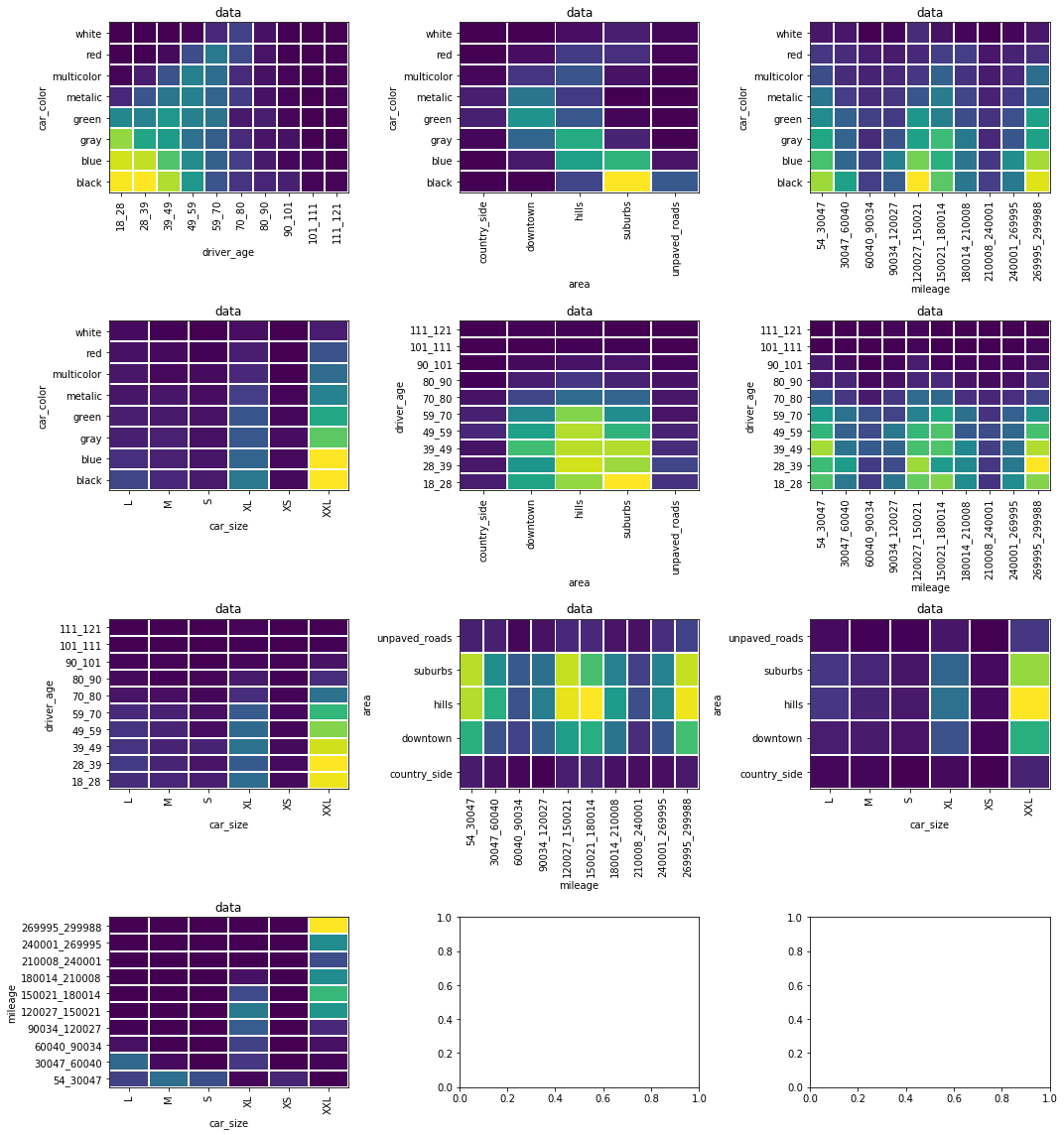# Correlation: mileage vs car_size¶

From the above plots it seems like there might be an interesting a correlation between mileage and car_size. Let's see what phik correlation is measured for this data.

In :
x, y = data[['mileage', 'car_size']].T.values

print('phik         =  %.2f'%phik.phik_from_array(x, y, num_vars=['x']))
print('significance = %.2f'%phik.significance_from_array(x, y, num_vars=['x']))

phik         =  0.77
significance = 49.22


Indeed there is a correlation between these variables and the correlation is also significant. To better understand the correlation, we can have a look at the signficance of excesses and deficits in the 2-dimensional contigency table, so-called "outlier significances".

In :
phik.outlier_significance_from_array(x, y, num_vars=['x'])

Out:
y L M S XL XS XXL
53.5_30047.0 6.882155 21.483476 18.076204 -8.209536 10.820863 -22.423985
30047.0_60040.5 20.034528 -0.251737 -3.408409 2.534277 -1.973628 -8.209536
60040.5_90033.9 1.627610 -3.043497 -2.265809 10.215936 -1.246784 -8.209536
90033.9_120027.4 -3.711579 -3.827278 -2.885475 12.999048 -1.638288 -7.185622
120027.4_150020.9 -7.665861 -6.173001 -4.746762 9.629145 -2.841508 -0.504521
150020.9_180014.4 -7.533189 -6.063786 -4.660049 1.559370 -2.785049 6.765549
180014.4_210007.8 -5.541940 -4.425929 -3.360023 -4.802787 -1.942469 10.520540
210007.8_240001.3 -3.496905 -2.745103 -2.030802 -5.850529 -1.100873 8.723925
240001.3_269994.8 -5.275976 -4.207164 -3.186534 -8.616464 -1.830944 13.303101
269994.8_299988.2 -8.014016 -6.458253 -4.973240 -12.868389 -2.989055 20.992824

The values displayed in the matrix are the signficiances of the outlier frequencies, i.e. a large value means that the measured frequency for that bin is significantly different from the expected frequency in that bin.

Let's visualise for easier interpretation.

In :
outlier_signifs = phik.outlier_significance_from_array(x, y, num_vars=['x'])

zvalues = outlier_signifs.values
xlabels = outlier_signifs.columns
ylabels = outlier_signifs.index
xlabel = 'x'
ylabel = 'y'

plot_correlation_matrix(zvalues, x_labels=xlabels, y_labels=ylabels,
x_label=xlabel,y_label=ylabel,
vmin=-5, vmax=5, title='outlier significance',
identity_layout=False, fontsize_factor=1.2)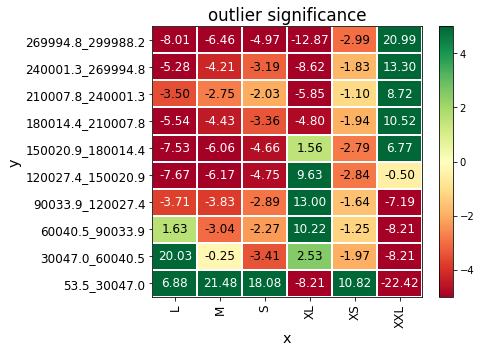# $\phi_k$ functions for dataframes¶

In our data we have 5 different columns, meaning we have to evaluate 4+3+2+1=10 pairs of variables for possible correlations. In a large dataset, with many different variables, this can easily become a cumbersome taks. Can we do this more efficient? yes! We have provided functions that work on dataframes, to allow you to calculate the phik correlation, significance and outlier significance for all different variable combinations at once.

The functions are by default available after import of the phik package.

# $\phi_k$ correlation matrix¶

Now let's start calculating the corrlation phik between pairs of variables.

Note that the original dataset is used as input, the binning of interval variables is done automatically.

In :
phik_overview = data.phik_matrix(interval_cols=interval_cols)
phik_overview

Out:
var2 area car_color car_size driver_age mileage
var1
area 1.000000 0.590456 0.000000 0.105506 0.000000
car_color 0.590456 1.000000 0.000000 0.389671 0.000000
car_size 0.000000 0.000000 1.000000 0.000000 0.768589
driver_age 0.105506 0.389671 0.000000 1.000000 0.000000
mileage 0.000000 0.000000 0.768589 0.000000 1.000000

When no interval colums are provided, the code makes an educated guess

In :
data.phik_matrix()

interval_cols not set, guessing: ['driver_age', 'mileage']

Out:
var2 area car_color car_size driver_age mileage
var1
area 1.000000 0.590456 0.000000 0.105506 0.000000
car_color 0.590456 1.000000 0.000000 0.389671 0.000000
car_size 0.000000 0.000000 1.000000 0.000000 0.768589
driver_age 0.105506 0.389671 0.000000 1.000000 0.000000
mileage 0.000000 0.000000 0.768589 0.000000 1.000000
In :
plot_correlation_matrix(phik_overview.values, x_labels=phik_overview.columns, y_labels=phik_overview.index,
vmin=0, vmax=1, color_map='Blues', title=r'correlation $\phi_K$', fontsize_factor=1.5,
figsize=(7,5.5))
plt.tight_layout()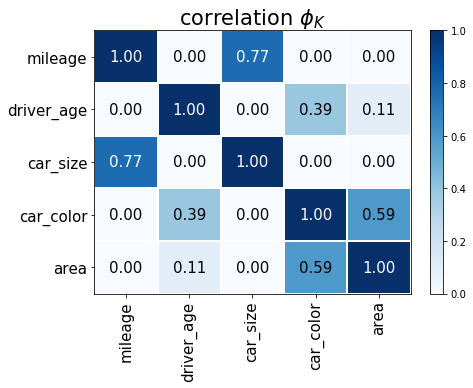# Global correlation: $g_k$¶

The global correlation coefficient is a measure of the total correlation of one variable to all other variables in the dataset. They give an indication of how well on variable can be modelled in terms of the other variables. A calculation of the global correlation coefficient is provided within the phik package.

In :
global_correlation, global_labels = data.global_phik(interval_cols=interval_cols)
for c, l in zip(global_correlation, global_labels):
print(l, c)

area 0.6057528003711345
car_color 0.6760317420050453
car_size 0.768588987856336
driver_age 0.41913005031633166
mileage 0.768588987856336

In :
plot_correlation_matrix(global_correlation, x_labels=[''], y_labels=global_labels,
vmin=0, vmax=1, figsize=(3.5,4),
color_map='Blues', title=r'$g_k$',
fontsize_factor=1.5)
plt.tight_layout()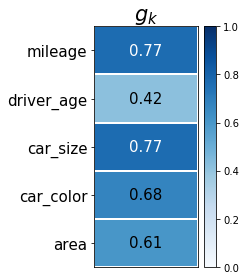# Statistical significance of the correlation: $Z$-score¶

When assessing correlations it is good practise to evaluate both the correlation and the significance of the correlation: a large correlation may be statistically insignificant, and vice versa a small correlation may be very significant. For instance, scipy.stats.pearsonr returns both the pearson correlation and the p-value. Similarly, the phik package offeres functionality the calculate a significance matrix. Significance is defined as:

$$Z = \Phi^{-1}(1-p)\ ;\quad \Phi(z)=\frac{1}{\sqrt{2\pi}} \int_{-\infty}^{z} e^{-t^{2}/2}\,{\rm d}t$$

Several corrections to the 'standard' p-value calculation are taken into account, making the method more rebust for low statistics and sparse data cases. The user is referred to our paper for more details.

As a result, the calculation may take a few seconds.

In :
significance_overview = data.significance_matrix(interval_cols=interval_cols)
significance_overview

Out:
var2 area car_color car_size driver_age mileage
var1
area 72.414143 37.597152 -0.292777 1.792994 -0.644337
car_color 37.597152 85.456758 -0.627259 19.823435 -0.636309
car_size -0.292777 -0.627259 69.041964 -0.539766 49.224630
driver_age 1.792994 19.823435 -0.539766 84.350588 -0.706470
mileage -0.644337 -0.636309 49.224630 -0.706470 91.208501
In :
plot_correlation_matrix(significance_overview.fillna(0).values, x_labels=significance_overview.columns,
y_labels=significance_overview.index, vmin=-5, vmax=5, title='significance',
usetex=False, fontsize_factor=1.5, figsize=(7,5.5))
plt.tight_layout()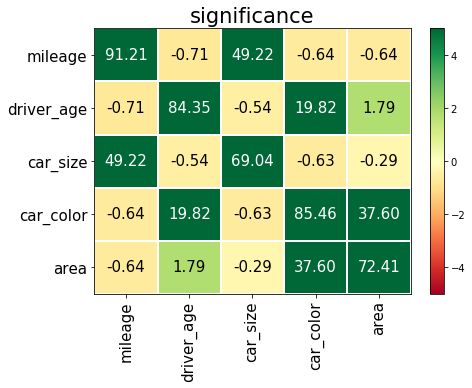# Outlier significance¶

The normal pearson correlation between two interval variables is easy to interpret. However, the phik correlation between two variables of mixed type is not always easy to interpret, especially when it concerns categorical veriables. Therefore, functionality is providided to detect "outliers": excesses and deficicts over the exptected frequencies in the contigency table of two varaibles.

### Example 1: car_color versus area¶

For the categorical variable pair car_color - area we measured:

$$\phi_k = 0.59 \, ,\quad\quad \mathrm{significance} = 37.6$$

Let's use the outlier signifiance functionality to gain a better understanding of the sigificance correlation between car color and area.

In :
c1 = 'car_color'
c0 = 'area'

In :
outlier_signifs, binning_dict = data[[c0,c1]].outlier_significance_matrix(retbins=True)
outlier_signifs

Out:
car_color black blue gray green metalic multicolor red white
area
country_side -5.636485 -5.277175 -1.688032 5.824664 6.506020 0.092630 -2.282290 -1.193468
downtown -16.444319 -8.209536 3.450521 14.176441 12.238785 2.582260 -3.863796 -4.400847
hills -8.209536 3.054306 9.244711 -2.097600 -3.022553 4.789665 2.695864 -1.092375
suburbs 19.533221 8.315558 -8.209536 -8.209536 -8.209536 -6.529846 1.667814 3.964555
unpaved_roads 13.043553 -1.776804 -6.095289 -5.532779 -4.566500 -3.829519 -1.339881 1.074386
In :
zvalues = outlier_signifs.values
xlabels = binning_dict[c1] if c1 in binning_dict.keys() else outlier_signifs.columns
ylabels = binning_dict[c0] if c0 in binning_dict.keys() else outlier_signifs.index
xlabel = c1
ylabel = c0

plot_correlation_matrix(zvalues, x_labels=xlabels, y_labels=ylabels,
x_label=xlabel,y_label=ylabel,
vmin=-5, vmax=5, title='outlier significance',
identity_layout=False, fontsize_factor=1.2)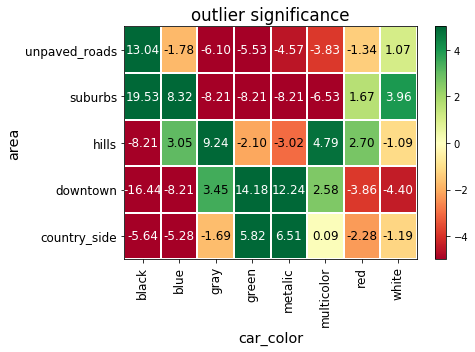The significance of each cell is expressed in terms of Z (one-sided).

Interesting, owners of a green car are more likely to live in the country side, and black cars are more likely to travel on unpaved roads!

### Example 2: mileage versus car_size¶

For the categorical variable pair mileage - car_size we measured:

$$\phi_k = 0.77 \, ,\quad\quad \mathrm{significance} = 46.3$$

Let's use the outlier signifiance functionality to gain a better understanding of this sigificance correlation between mileage and car size.

In :
c0 = 'mileage'
c1 = 'car_size'

tmp_interval_cols = ['mileage']

In :
outlier_signifs, binning_dict = data[[c0,c1]].outlier_significance_matrix(interval_cols=tmp_interval_cols,
retbins=True)
outlier_signifs

Out:
car_size L M S XL XS XXL
53.5_30047.0 6.882155 21.483476 18.076204 -8.209536 10.820863 -22.423985
30047.0_60040.5 20.034528 -0.251737 -3.408409 2.534277 -1.973628 -8.209536
60040.5_90033.9 1.627610 -3.043497 -2.265809 10.215936 -1.246784 -8.209536
90033.9_120027.4 -3.711579 -3.827278 -2.885475 12.999048 -1.638288 -7.185622
120027.4_150020.9 -7.665861 -6.173001 -4.746762 9.629145 -2.841508 -0.504521
150020.9_180014.4 -7.533189 -6.063786 -4.660049 1.559370 -2.785049 6.765549
180014.4_210007.8 -5.541940 -4.425929 -3.360023 -4.802787 -1.942469 10.520540
210007.8_240001.3 -3.496905 -2.745103 -2.030802 -5.850529 -1.100873 8.723925
240001.3_269994.8 -5.275976 -4.207164 -3.186534 -8.616464 -1.830944 13.303101
269994.8_299988.2 -8.014016 -6.458253 -4.973240 -12.868389 -2.989055 20.992824

Note that the interval variable mileage is binned automatically in 10 uniformly spaced bins!

In :
zvalues = outlier_signifs.values
xlabels = outlier_signifs.columns
ylabels = outlier_signifs.index
xlabel = c1
ylabel = c0

plot_correlation_matrix(zvalues, x_labels=xlabels, y_labels=ylabels,
x_label=xlabel,y_label=ylabel,
vmin=-5, vmax=5, title='outlier significance',
identity_layout=False, fontsize_factor=1.2)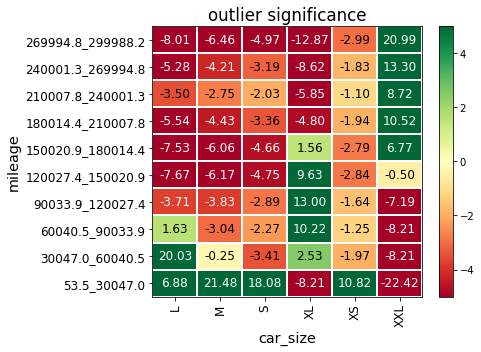# Correlation report¶

A full correlation report can be created automatically for a dataset by pairwise evalation of all correlations, significances and outlier significances.

Note that for a dataset with many different columns the number of outlier significances plots can grow large very rapidly. Therefore, the feature is implemented to only evaluate outlier significances for those variable pairs with a significance and correlation larger than the given thresholds.

In :
from phik import report
rep = report.correlation_report(data, significance_threshold=3, correlation_threshold=0.5)

interval_cols not set, guessing: ['driver_age', 'mileage']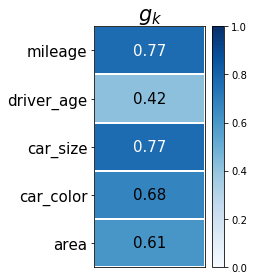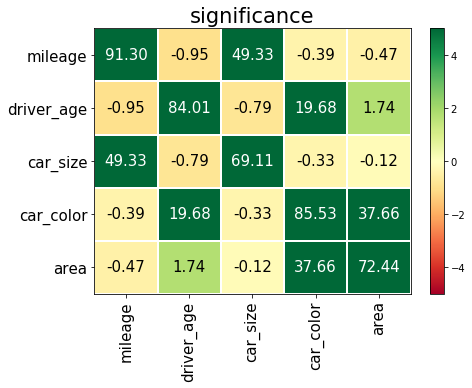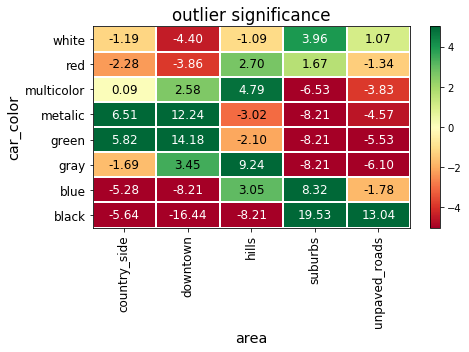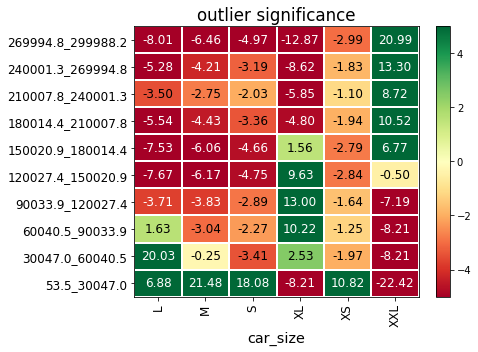# Recap¶

To summarize, the main functions in the phik correlation package working on a dataframe are:

• df[twocols].hist2d()
• df.phik_matrix()
• df.global_phik()
• df.significance_matrix()
• df.outlier_significance_matrix() -- note: on a dataframe with exactly 2 columns
• df.outlier_significance_matrices()
In :
data[['driver_age', 'mileage']].hist2d()

interval_cols not set, guessing: ['driver_age', 'mileage']

Out:
mileage 1 2 3 4 5 6 7 8 9 10
driver_age
1 54.0 30.0 13.0 29.0 57.0 61.0 36.0 11.0 36.0 61.0
2 51.0 41.0 13.0 17.0 64.0 41.0 35.0 12.0 30.0 75.0
3 65.0 29.0 21.0 24.0 53.0 54.0 35.0 14.0 27.0 66.0
4 49.0 23.0 17.0 30.0 50.0 54.0 21.0 17.0 25.0 53.0
5 41.0 28.0 19.0 15.0 33.0 45.0 27.0 11.0 23.0 39.0
6 21.0 12.0 5.0 12.0 26.0 25.0 10.0 8.0 10.0 15.0
7 7.0 8.0 2.0 3.0 8.0 9.0 4.0 2.0 3.0 10.0
8 5.0 2.0 0.0 1.0 6.0 1.0 1.0 1.0 1.0 3.0
9 0.0 0.0 0.0 0.0 1.0 0.0 0.0 0.0 0.0 1.0
10 0.0 0.0 0.0 1.0 1.0 0.0 0.0 0.0 0.0 0.0
In :
data.phik_matrix()

interval_cols not set, guessing: ['driver_age', 'mileage']

Out:
var2 area car_color car_size driver_age mileage
var1
area 1.000000 0.590456 0.000000 0.105506 0.000000
car_color 0.590456 1.000000 0.000000 0.389671 0.000000
car_size 0.000000 0.000000 1.000000 0.000000 0.768589
driver_age 0.105506 0.389671 0.000000 1.000000 0.000000
mileage 0.000000 0.000000 0.768589 0.000000 1.000000
In :
data.global_phik()

interval_cols not set, guessing: ['driver_age', 'mileage']

Out:
(array([[0.6057528 ],
[0.67603174],
[0.76858899],
[0.41913005],
[0.76858899]]),
array(['area', 'car_color', 'car_size', 'driver_age', 'mileage'],
dtype=object))
In :
data.significance_matrix()

interval_cols not set, guessing: ['driver_age', 'mileage']

Out:
var2 area car_color car_size driver_age mileage
var1
area 72.414149 37.598215 -0.285072 1.833756 -0.632824
car_color 37.598215 85.465972 -0.636051 19.817000 -0.577392
car_size -0.285072 -0.636051 69.049134 -0.496392 49.232915
driver_age 1.833756 19.817000 -0.496392 84.343356 -0.684187
mileage -0.632824 -0.577392 49.232915 -0.684187 91.236359
In :
data[['area', 'mileage']].outlier_significance_matrix()

interval_cols not set, guessing: ['mileage']

Out:
53.5_30047.0 30047.0_60040.5 60040.5_90033.9 90033.9_120027.4 120027.4_150020.9 150020.9_180014.4 180014.4_210007.8 210007.8_240001.3 240001.3_269994.8 269994.8_299988.2
area
country_side -0.178204 0.192584 0.099073 -1.818924 0.103907 0.888225 0.251480 1.491532 0.016080 -0.869129
downtown 0.625586 -2.141106 0.541785 0.931452 -0.926177 0.582585 0.917301 -0.729204 -0.978248 0.610302
hills -0.826807 1.346029 -0.841090 0.008498 0.071356 1.028940 0.107051 0.080217 -0.397630 -0.904236
suburbs 0.972621 0.098196 0.712079 -0.059975 0.975536 -1.759184 -0.716170 -0.603623 0.059615 -0.050745
unpaved_roads -1.469438 0.518041 -0.915745 -0.475005 -0.679357 -0.545607 -0.808321 0.901333 2.175620 1.339934
In :
os_matrices = data.outlier_significance_matrices()

interval_cols not set, guessing: ['driver_age', 'mileage']

In :
os_matrices.keys()

Out:
dict_keys(['car_color:driver_age', 'car_color:area', 'car_color:mileage', 'car_color:car_size', 'driver_age:area', 'driver_age:mileage', 'driver_age:car_size', 'area:mileage', 'area:car_size', 'mileage:car_size'])
In :
os_matrices['car_color:mileage']

Out:
53.5_30047.0 30047.0_60040.5 60040.5_90033.9 90033.9_120027.4 120027.4_150020.9 150020.9_180014.4 180014.4_210007.8 210007.8_240001.3 240001.3_269994.8 269994.8_299988.2
car_color
black 0.366425 1.387093 -1.320194 -1.274558 1.908010 -0.880578 -1.260293 -0.498607 -0.449794 0.492858
blue -0.409760 -1.676495 -0.515491 1.969650 0.474818 -1.260466 -0.505987 -0.575699 1.675451 0.601026
gray 0.267888 -0.338430 -1.148378 0.048682 -0.238285 1.522769 1.282298 -0.812279 -0.589854 -0.887127
green -0.195906 0.399471 1.306739 -0.918723 0.373229 -0.859866 -0.862544 0.772096 -0.169912 0.471057
metalic 0.697184 -0.385806 0.672620 0.205599 -1.543500 1.168147 0.249471 0.513459 -0.174870 -0.952009
multicolor -0.478862 -0.591987 0.893783 0.050843 -2.104857 0.994621 0.109020 0.578461 -0.506460 1.287415
red -0.551106 0.548949 1.370043 -0.050064 -1.822748 0.055521 2.208175 0.921955 0.219336 -1.556492
white -0.055025 1.219026 -0.596627 -0.427730 1.888206 -0.471175 -0.851352 -0.393054 -0.697834 -0.323899